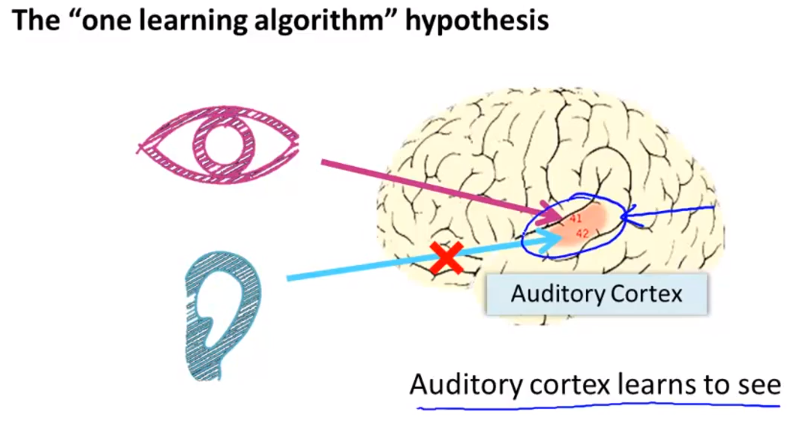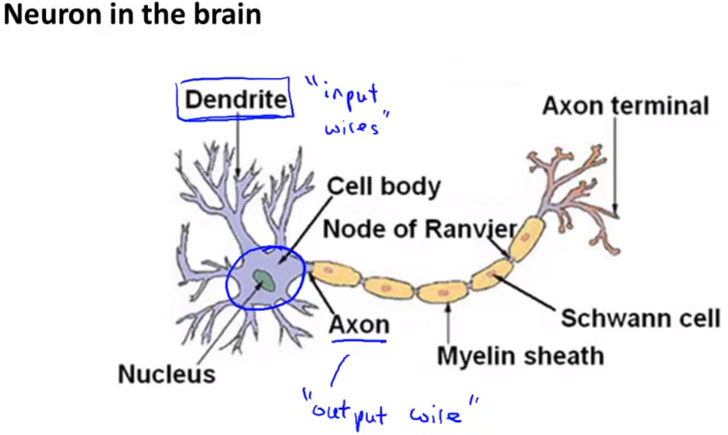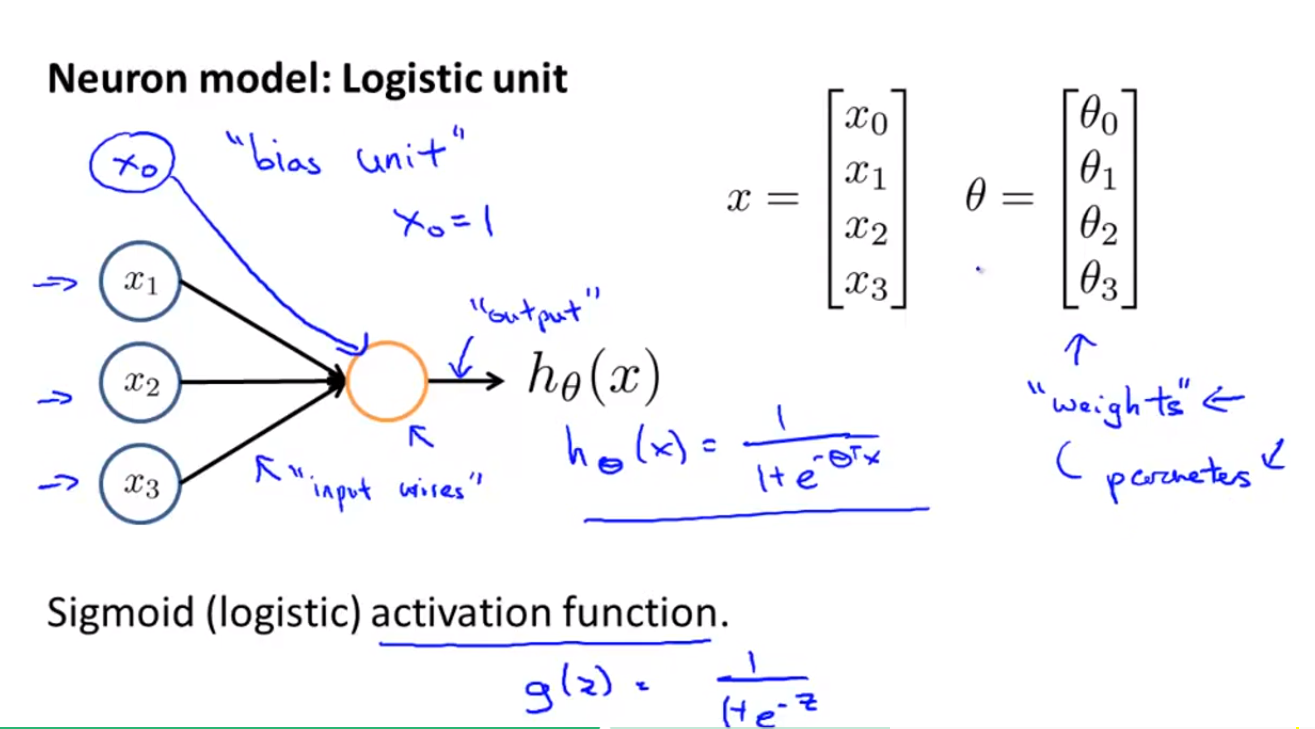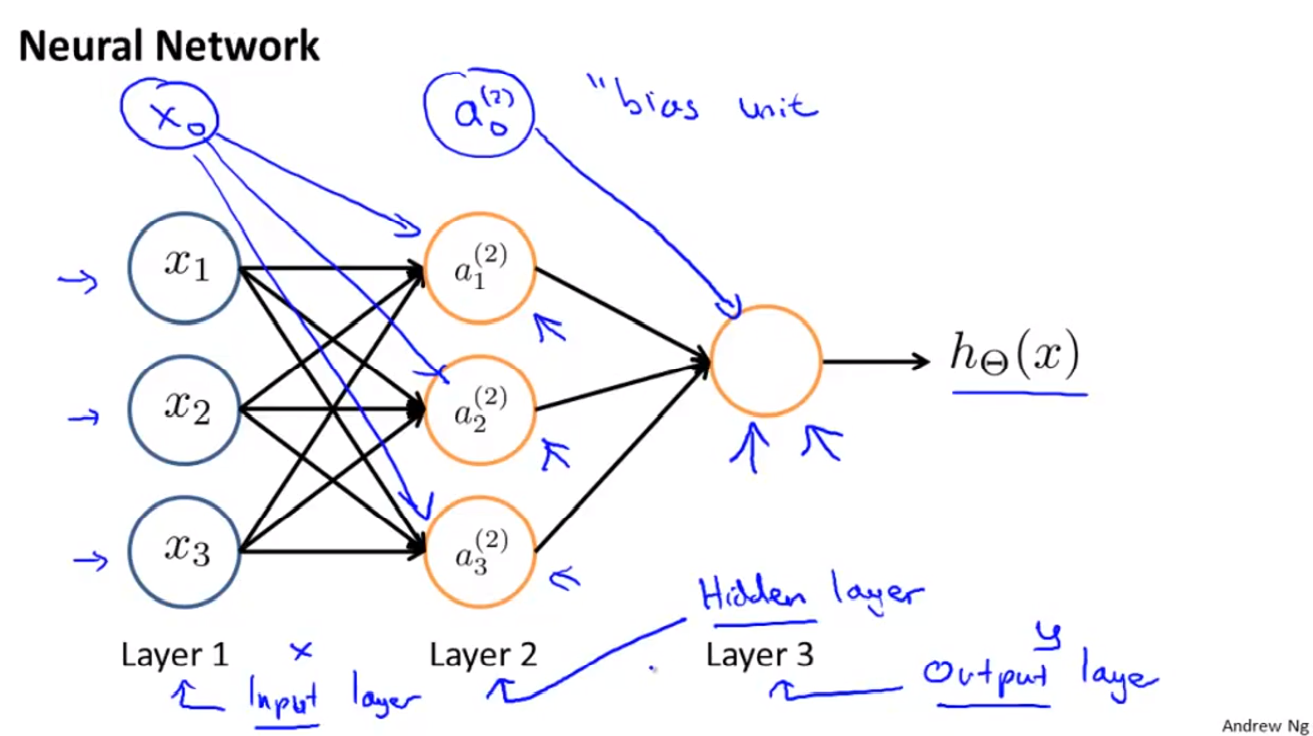ZhouYongyi's Personal Blog# 吴恩达《机器学习》课程学习笔记（六）—— 神经网络的表达

## 一、非线性假设（Non-linear Hypotheses）

\begin{align*}& g(\theta_0 + \theta_1x_1^2 + \theta_2x_1x_2 + \theta_3x_1x_3 \newline& + \theta_4x_2^2 + \theta_5x_2x_3 \newline& + \theta_6x_3^2 )\end{align*}

## 二、神经元和大脑（Neurons and the Brain）## 三、模型表示（Model Representation）

### 1、简单表达$\begin{bmatrix}x_0 \newline x_1 \newline x_2 \newline \end{bmatrix}\rightarrow\begin{bmatrix}\ \ \ \newline \end{bmatrix}\rightarrow h_\theta(x)$

\begin{align*}& a_i^{(j)} = \text{第j层的第i个激活单元} \newline& \Theta^{(j)} = \text{控制从第j层到j+1层的映射函数的权重矩阵}\end{align*}

$\begin{bmatrix}x_0 \newline x_1 \newline x_2 \newline x_3\end{bmatrix}\rightarrow\begin{bmatrix}a_1^{(2)} \newline a_2^{(2)} \newline a_3^{(2)} \newline \end{bmatrix}\rightarrow h_\theta(x)$

\begin{align*} a_1^{(2)} = g(\Theta_{10}^{(1)}x_0 + \Theta_{11}^{(1)}x_1 + \Theta_{12}^{(1)}x_2 + \Theta_{13}^{(1)}x_3) \newline a_2^{(2)} = g(\Theta_{20}^{(1)}x_0 + \Theta_{21}^{(1)}x_1 + \Theta_{22}^{(1)}x_2 + \Theta_{23}^{(1)}x_3) \newline a_3^{(2)} = g(\Theta_{30}^{(1)}x_0 + \Theta_{31}^{(1)}x_1 + \Theta_{32}^{(1)}x_2 + \Theta_{33}^{(1)}x_3) \newline h_\Theta(x) = a_1^{(3)} = g(\Theta_{10}^{(2)}a_0^{(2)} + \Theta_{11}^{(2)}a_1^{(2)} + \Theta_{12}^{(2)}a_2^{(2)} + \Theta_{13}^{(2)}a_3^{(2)}) \newline \end{align*}

### 2、向量形式表达

\begin{align*}a_1^{(2)} = g(z_1^{(2)}) \newline a_2^{(2)} = g(z_2^{(2)}) \newline a_3^{(2)} = g(z_3^{(2)}) \newline \end{align*}

$z_k^{(2)} = \Theta_{k,0}^{(1)}x_0 + \Theta_{k,1}^{(1)}x_1 + \cdots + \Theta_{k,n}^{(1)}x_n$

\begin{align*}x = \begin{bmatrix}x_0 \newline x_1 \newline\cdots \newline x_n\end{bmatrix} &z^{(j)} = \begin{bmatrix}z_1^{(j)} \newline z_2^{(j)} \newline\cdots \newline z_n^{(j)}\end{bmatrix}\end{align*}

$z^{(j)} = \Theta^{(j-1)}a^{(j-1)}\space\space\space\space\space\space(3.1)$

$a^{(j)} = g(z^{(j)})\space\space\space\space\space\space(3.2)$

$z^{(j+1)} = \Theta^{(j)}a^{(j)}$

$h_\Theta(x) = a^{(j+1)} = g(z^{(j+1)}) \space\space\space\space\space\space(3.3)$

### 3、例子

\begin{align*}\begin{bmatrix}x_0 \newline x_1 \newline x_2\end{bmatrix} \rightarrow\begin{bmatrix}g(z^{(2)})\end{bmatrix} \rightarrow h_\Theta(x)\end{align*}

$\Theta^{(1)} =\begin{bmatrix}-30 & 20 & 20\end{bmatrix}$

\begin{align*}& h_\Theta(x) = g(-30 + 20x_1 + 20x_2) \newline \newline & x_1 = 0 \ \ and \ \ x_2 = 0 \ \ then \ \ g(-30) \approx 0 \newline & x_1 = 0 \ \ and \ \ x_2 = 1 \ \ then \ \ g(-10) \approx 0 \newline & x_1 = 1 \ \ and \ \ x_2 = 0 \ \ then \ \ g(-10) \approx 0 \newline & x_1 = 1 \ \ and \ \ x_2 = 1 \ \ then \ \ g(10) \approx 1\end{align*}

\begin{align*}AND:\newline\Theta^{(1)} &=\begin{bmatrix}-30 & 20 & 20\end{bmatrix} \newline NOR:\newline\Theta^{(1)} &= \begin{bmatrix}10 & -20 & -20\end{bmatrix} \newline OR:\newline\Theta^{(1)} &= \begin{bmatrix}-10 & 20 & 20\end{bmatrix} \newline\end{align*}

## 四、多类型分类（Multiclass Classification）

\begin{align*}\begin{bmatrix}x_0 \newline x_1 \newline x_2 \newline\cdots \newline x_n\end{bmatrix} \rightarrow\begin{bmatrix}a_0^{(2)} \newline a_1^{(2)} \newline a_2^{(2)} \newline\cdots\end{bmatrix} \rightarrow\begin{bmatrix}a_0^{(3)} \newline a_1^{(3)} \newline a_2^{(3)} \newline\cdots\end{bmatrix} \rightarrow \cdots \rightarrow\begin{bmatrix}h_\Theta(x)_1 \newline h_\Theta(x)_2 \newline h_\Theta(x)_3 \newline h_\Theta(x)_4 \newline\end{bmatrix} \rightarrow\end{align*}

$h_\Theta(x) =\begin{bmatrix}0 \newline 0 \newline 1 \newline 0 \newline\end{bmatrix}$

$h_\Theta(x) =\begin{bmatrix}0.2 \newline 0.8 \newline 0.2 \newline 0 \newline\end{bmatrix}$

## 五、第三次编程作业##### Zhao##### zhouyongyi

ヾ(◍°∇°◍)ﾉﾞ加油

$${}$$